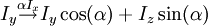# Drawing mathematical equations

(diff) ←Older revision | Current revision (diff) | Newer revision→ (diff)

Place latex code between  tags

Here is an example

$I_y \stackrel{\alpha I_x}{\rightarrow} I_y \cos(\alpha) + I_z\sin(\alpha)$


which gives$I_y \stackrel{\alpha I_x}{\rightarrow} I_y \cos(\alpha) + I_z\sin(\alpha)$

Many more examples are available here